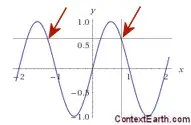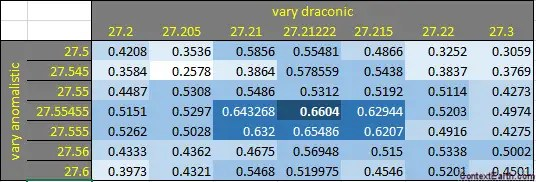# ENSO and Tidal SLH – A Biennial Connection

[mathjax]It’s becoming abundantly clear that ENSO is driven by lunisolar mechanisms, especially true considering that we use the preferred models describing sloshing of water volumes — the Mathieu equation and the delay differential equation. What’s more, given the fact that the ENSO model works so well, one can guess that a connection between ENSO and lunar tidal forces should carry over to models for historical sea-level height (SLH) tidal data.

From my preliminary work analyzing SLH tidal data in 2014, I found an intriguing pattern relating to the same biennial pattern observed in the ENSO model. An extract from that post is reproduced below:

“The idea, related to delay differential equations, is to determine if it is at all possible to model at least part of ENSO (through the SOI) with data from a point in time in the tidal record with a compensated point from the past. This essentially models the effect of the current wave being cancelled partially by the reflection of a previous wave.

This is essentially suggesting that $$SOI = k f(t) – k f(t-Delta t)$$ where f(t) is the tidal record and k is a constant.

After some experimenting, a good fit is obtained when the current tidal data is set to 3 months ago, and the prior data is taken from 26 months in the past. To model the negative of ENSO, the 3-month old data is subtracted from the 26-month old data, Figure 1:”Fig 1: Model of ENSO uses Tidal Gauge readings from Sydney Harbor.

This observation suggests a biennial pattern whereby high correlations in the time series occur for events observed close to two-years apart, with the difference taken up by the ENSO signal. The latter is at least partly due to the inverted barometer effect on SLH.

The sensitivity of the biennial effect is shown in the following contour diagram, where the peak CC is indicated and a slanted line showing the 2-year differencing points (table of the correlation coefficients here)Focussing on the mechanisms for the correlation, the predictor can be reformulated as$f(t) = f(t-Delta t) + SOI / k$

or that the 2-year prior SLH reading, with the addition of the SOI signal, will accurately represent the current SLH reading subject to a ~3 month lag.

My thinking is that this correlation can only occur if there is an actual biennial signal in the SLH itself. We can mathematically conjecture what is happening with the two year differencing by assuming that the SLH has the same height offset whenever pairs of points are separated by two years. You can see this in the following figure:This brings up an interesting question as to why this biennial signal apparently can’t be detected via conventional means. A likely guess is because it gets obscured by the ENSO signal. And since the ENSO signal has never been adequately modeled, no one has an inkling that a biennial signal coexists in the mix. Yet this unconventional delay differencing approach (incidentally discovered with the help of machine learning) is able to discern it with a strong statistical significance.

The gist of this discovery is that a biennial tidal SLH motion exists and likely contributes (or at least identifies) the nonlinear biennial modulation driving the ENSO model described in previous posts.

Summary:

ENSO has elements of an annual teeter-totter. If you look through the research literature, you will find numerous references to a hypothesized behavior that one annual peak is followed by a lesser peak the next year. Yet, no evidence that this strict biennial cycle is evidence in the data — it’s more of a hand-wavy physical argument that this can or should occur.

The way to model this teeter-totter behavior is via Mathieu equations and delay differential equations. Both of these provide a kind of non-linear modulation that can sustain a biennial feedback mechanism.

The other ingredient is a forcing mechanism. The current literature appears to agree that this is due to prevailing wind bursts, which to me seems intuitive but doesn’t answer what forces the wind in the first place. As it turns out, only two parameters are needed to force the DiffEq and these align precisely with the primary lunar cycles that govern transverse and longitudinal directional momentum, the draconic and anomalistic months.

The ENSO model turns into a metrology tool

Note the following table and how the draconic and anomalistic values zone in on the true value when correlating model against data:Fitting the DiffEq values progressively away from the true value for the lunar tidal cycle results in a smaller correlation coefficient.

Having one of these values align may be coincidence, but having both combine with that kind of resolution is telling. The biennial connection to SLH tidal measurements is substantiation that a biennial modulation is intrinsic to the physical process.

## 6 thoughts on “ENSO and Tidal SLH – A Biennial Connection”

1. Pingback: Scaling El Nino | context/Earth

2.tours of turkey

Valid for travel from 11 January – 20 June 2019.

Like

3.geoenergymath

Like